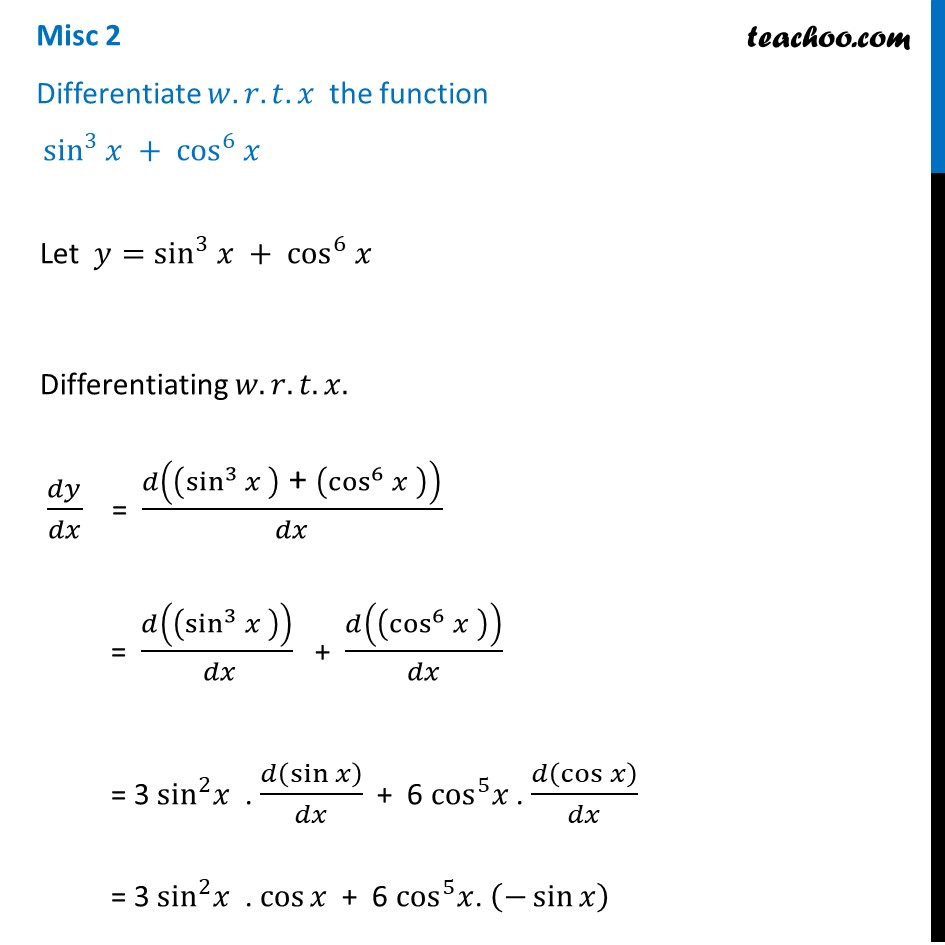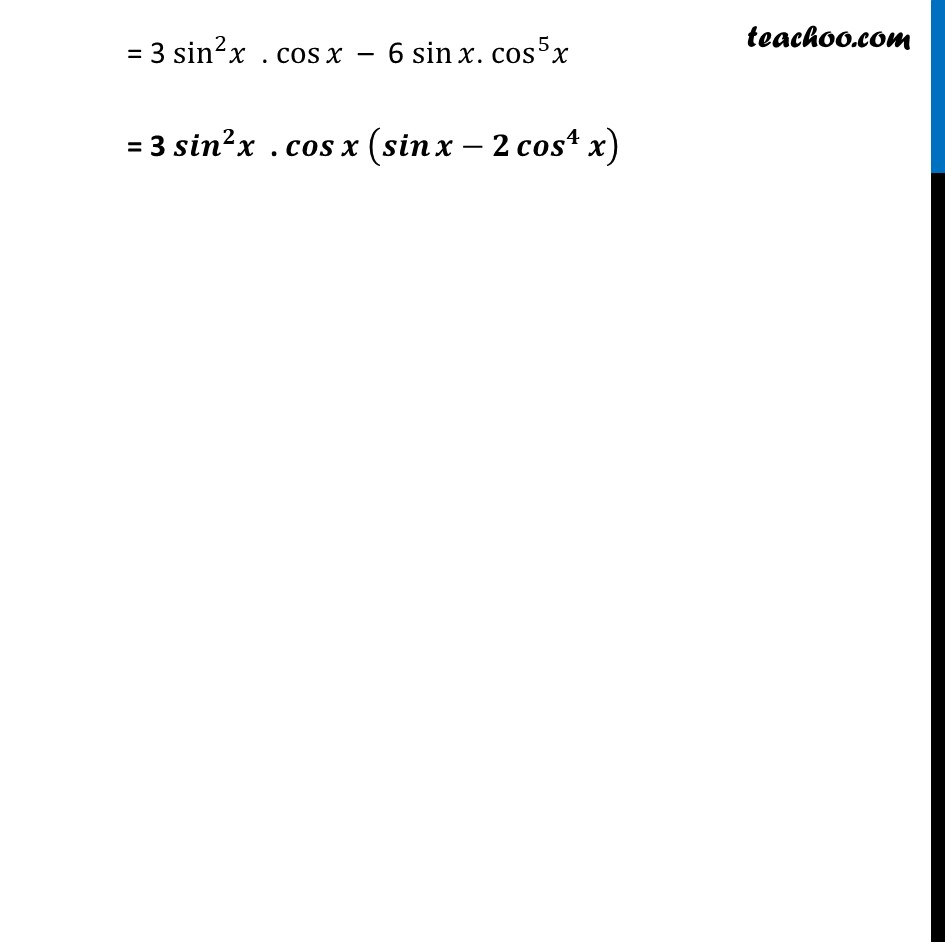Miscellaneous

Chapter 5 Class 12 Continuity and Differentiability
Serial order wiseLearn in your speed, with individual attention - Teachoo Maths 1-on-1 Class

### Transcript

Misc 2 Differentiate 𝑤.𝑟.𝑡. 𝑥 the function sin^3 𝑥 + cos^6 𝑥 Let 𝑦=sin^3 𝑥 + cos^6 𝑥 Differentiating 𝑤.𝑟.𝑡.𝑥. 𝑑𝑦/𝑑𝑥 = 𝑑((sin^3 𝑥 )" + " (cos^6 𝑥" " ))/𝑑𝑥 = 𝑑((sin^3 𝑥 ))/( 𝑑𝑥) + 𝑑((cos^6 𝑥" " ))/𝑑𝑥 = 3 sin^2 𝑥 . 𝑑(sin⁡𝑥 )/𝑑𝑥 + 6 cos^5 𝑥" ". 𝑑(cos⁡𝑥 )/𝑑𝑥 = 3 sin^2 𝑥 . cos⁡𝑥 + 6 cos^5 𝑥. (−sin⁡𝑥 ) = 3 sin^2 𝑥 . cos⁡𝑥 – 6 sin⁡𝑥. cos^5 𝑥 = 3 〖𝒔𝒊𝒏〗^𝟐 𝒙 . 𝒄𝒐𝒔⁡𝒙 (𝒔𝒊𝒏⁡𝒙−𝟐 〖𝒄𝒐𝒔〗^𝟒⁡𝒙 )